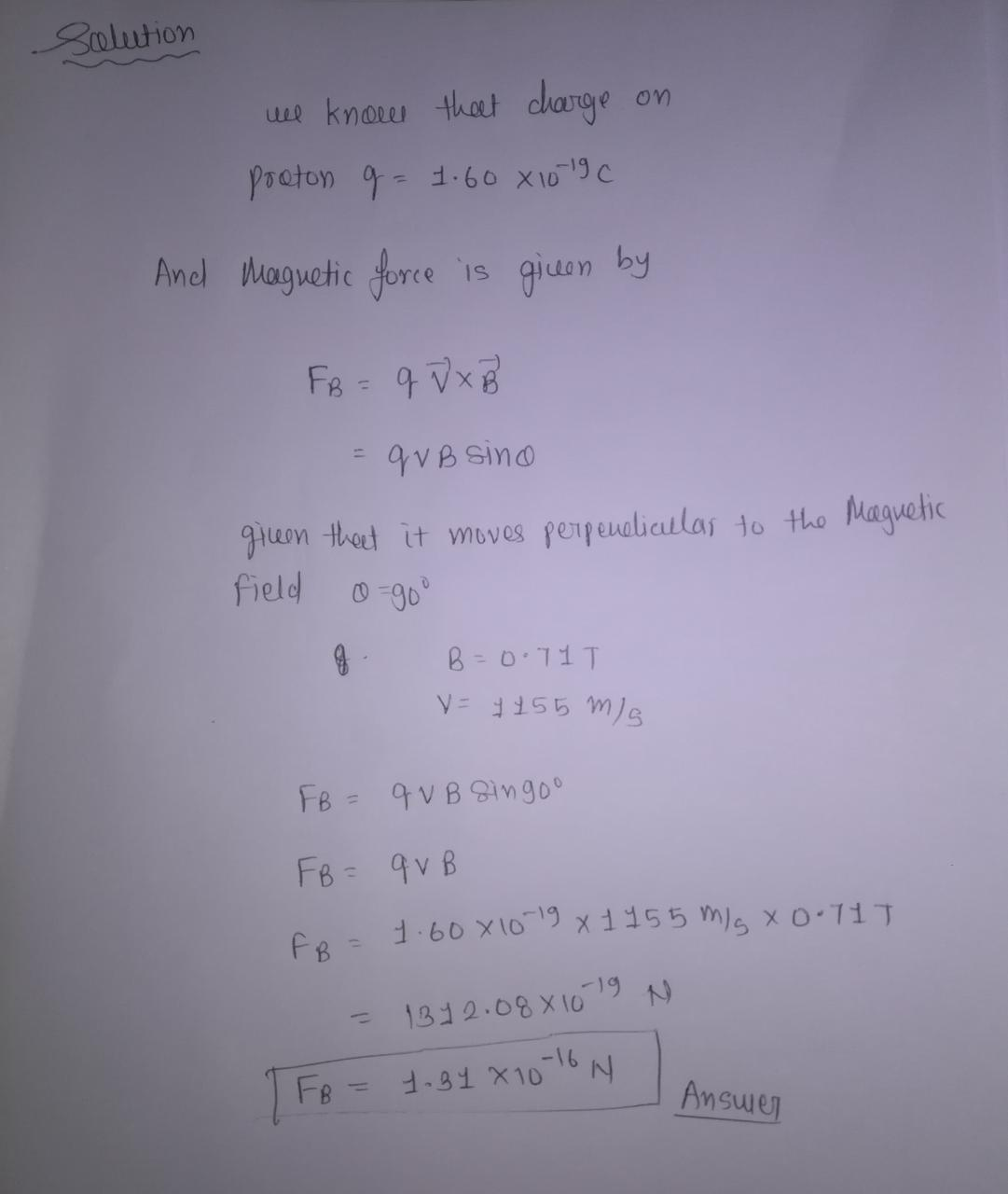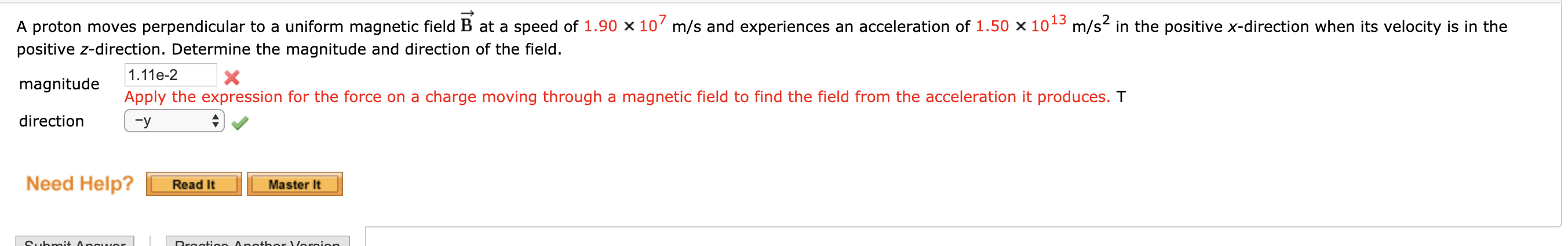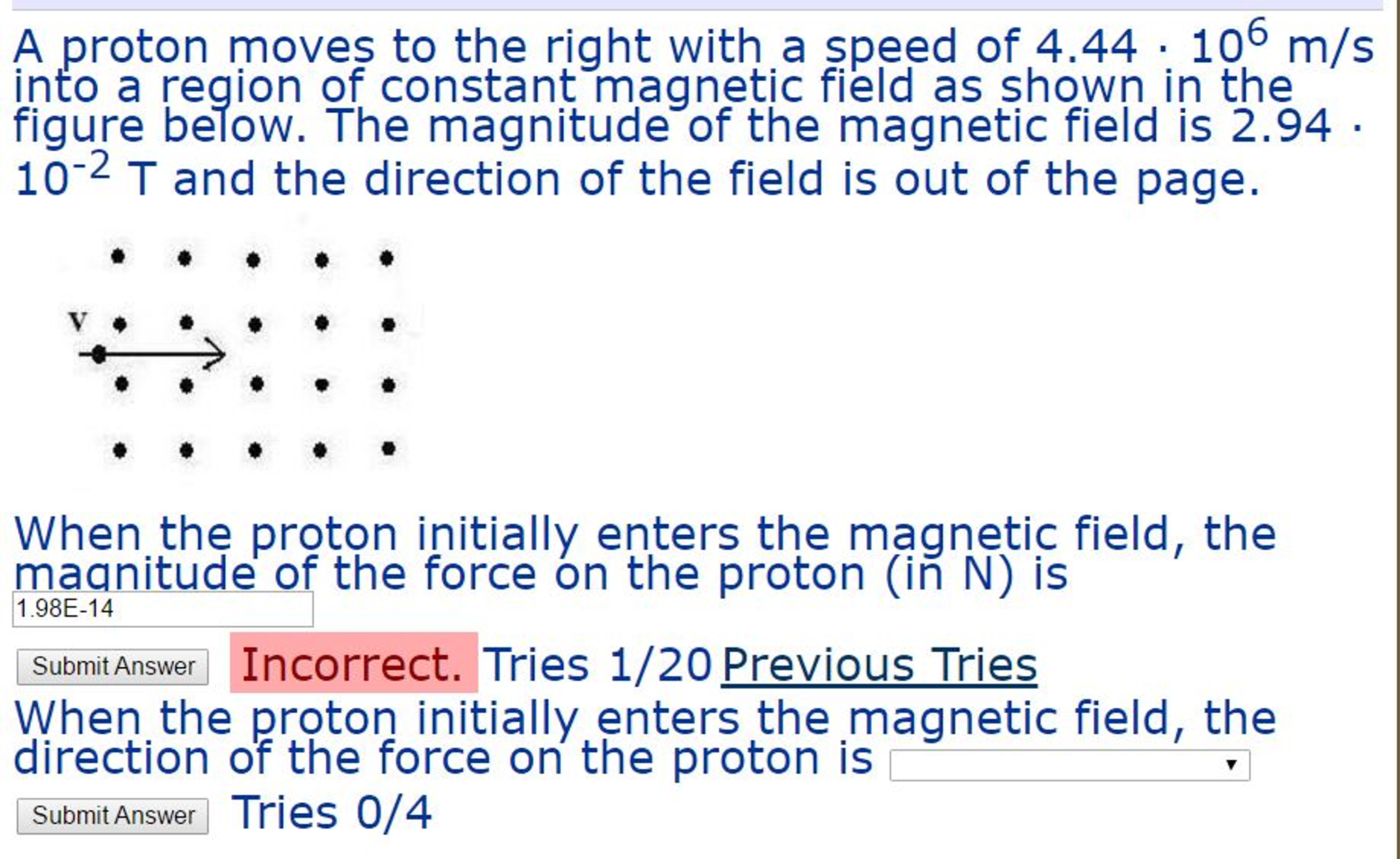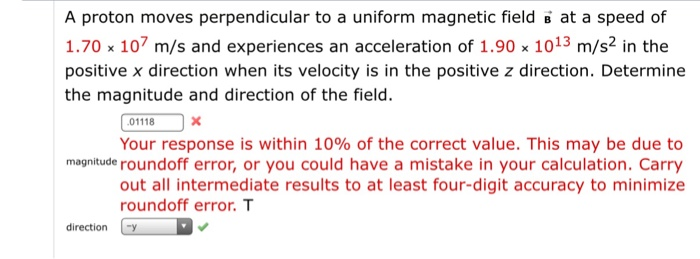Question

# A proton moves at a speed of 1155 m/s in a direction perpendicular to a magnetic...

A proton moves at a speed of 1155 m/s in a direction perpendicular to a magnetic field with a magnitude of 0.71 T. What is the magnitude of the magnetic force on the proton?g

#### Earn Coins

Coins can be redeemed for fabulous gifts.

Similar Homework Help Questions
• ### A proton moves perpendicular to a uniform magnetic field B at a speed of 1.90 x...A proton moves perpendicular to a uniform magnetic field B at a speed of 1.90 x 107 m/s and experiences an acceleration of 1.50 x 1013 m/s2 in the positive x-direction when its velocity is in the positive z-direction. Determine the magnitude and direction of the field. 1.11e-2 magnitude Apply the expression for the force on a charge moving through a magnetic field to find the field from the acceleration it produces. T direction - Need Help? Read It Master...

• ### A proton moves perpendicular to a uniform magnetic field B with arrow at a speed of...

A proton moves perpendicular to a uniform magnetic field B with arrow at a speed of 2.10 107 m/s and experiences an acceleration of 1.80 1013 m/s2 in the positive x-direction when its velocity is in the positive z-direction. Determine the magnitude and direction of the field. magnitude =

• ### A proton moves to the right with a speed of 4.44 middot 10^6 m/s into a...A proton moves to the right with a speed of 4.44 middot 10^6 m/s into a region of constant magnetic field as shown in the figure below. The magnitude of the magnetic field is 2.94 middot 10^-2 T and the direction of the field is out of the page. When the proton initially enters the magnetic field, the magnitude of the force on the proton (in N) is When the proton initially enters the magnetic field, the direction of the...

• ### A proton moves perpendicular to a uniform magnetic field B at a speed of 1.70 times...A proton moves perpendicular to a uniform magnetic field B at a speed of 1.70 times 10^7 m/s and experiences an acceleration of 1.90 times 10^13 m/s^2 in the positive x direction when its velocity is in the positive z direction. Determine the magnitude and direction of the field. Your response is within 10% of the correct value. This may be due to roundoff error, or you could have a mistake in your calculation. Carry out all intermediate results to...

• ### A proton moving in the positive x direction with a speed of 9.1 105 m/s experiences...

A proton moving in the positive x direction with a speed of 9.1 105 m/s experiences zero magnetic force. When it moves in the positive y direction it experiences a force of 1.8 10-13 N that points in the positive z direction. Determine the magnitude and direction of the magnetic field. magnitude     T

• ### A proton moves in a circle with a speed of 430 m/s through a uniform magnetic...

A proton moves in a circle with a speed of 430 m/s through a uniform magnetic field of 2.0 T directed perpendicular to the circular path. 1) What is the orbital radius of the proton? (Express your answer using two significant figures.) (units in micro m)

• ### A proton moves to the left with a speed of 1.25 · 106 m/s into a...

A proton moves to the left with a speed of 1.25 · 106 m/s into a region of constant magnetic field as shown in the figure below. The magnitude of the magnetic field is 1.91 · 10-2 T and the direction of the field is into the page. When the proton initially enters the magnetic field, the magnitude of the force on the proton (in N) is A: 2.160×10-15 B: 2.872×10-15 C: 3.820×10-15 D: 5.081×10-15 E: 6.757×10-15 F: 8.987×10-15 G:...

• ### An electron moves at a speed of 470 m/s perpendicular to the direction of a uniform...

An electron moves at a speed of 470 m/s perpendicular to the direction of a uniform magnetic field of 0.9 T. What is the radius of the electron's circular orbit in units of nanometers (1 m = 109 nm)?

• ### 1) An electron moves with a speed of 4.7 x 104 m/s perpendicular to a uniform...

1) An electron moves with a speed of 4.7 x 104 m/s perpendicular to a uniform magnetic field of 0.40 T. What is the magnitude of the magnetic force on the electron?

• ### A metal bar 1.5 m in length moves in a direction perpendicular to a uniform magnetic...

A metal bar 1.5 m in length moves in a direction perpendicular to a uniform magnetic field of magnitude 0.85T with a speed of 2.2 m/s. Determine the magnitude of the induced electric field in the metal bar.